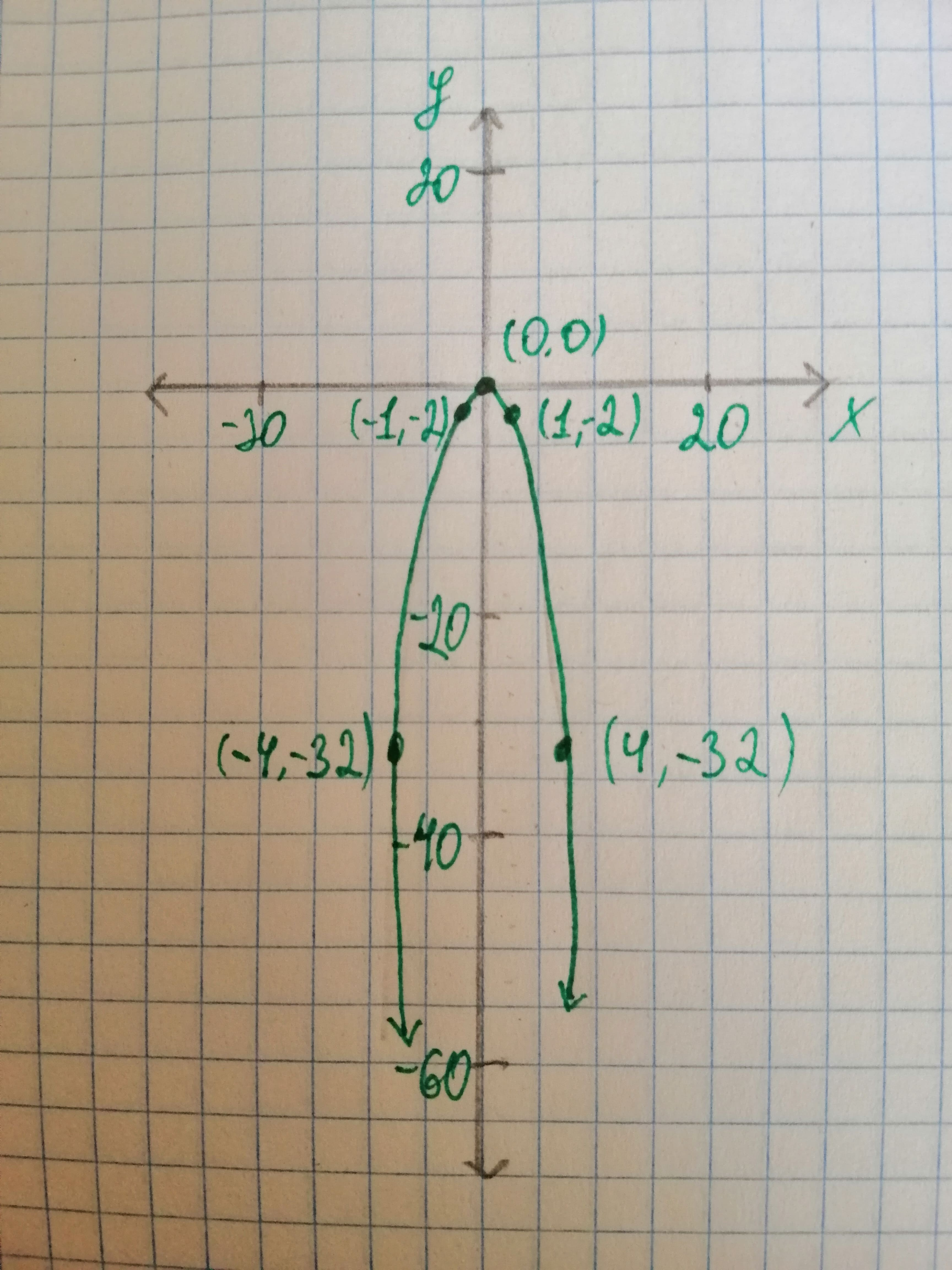# Determine which of the following functionsf(x)=cx, g(x)=cx^{2}, h(x)=csqrt{|x|}, text{and} r(x)= frac{c}{x}midtlinjeg 2021-02-02 Answered

Determine which of the following functions can be used to model the data and determine the value of the constant c that will make the function fit the data in the table. $\begin{array}{|cccccc|}\hline x& -4& -1& 0& 1& 4\\ y& -32& -2& 0& -2& -32\\ \hline\end{array}$

You can still ask an expert for help

• Questions are typically answered in as fast as 30 minutes

Solve your problem for the price of one coffee

• Math expert for every subject
• Pay only if we can solve itSadie Eaton

Consider the given function and the table. Now, with the help of the values given in the table, plot a graph as,Here, it can be seen that by plotting the data, the obtained graph represents a parabola. Therefore, the function which represents the data table is $g\left(x\right)=c{x}^{2}$. The point is on the graph. So, substitute the point, , in $g\left(x\right)=c{x}^{2}$.
$-32=c{\left(-4\right)}^{2}$
$-32=c\left(16\right)$
$\frac{-32}{16}=c$ Solving further, So, Hence, the function that can be used to model the data is $g\left(x\right)=c{x}^{2}$ and the value of constant is .